# Table 2 Summary of significance tests for combining different estimates from m imputed datasets after MI

Estimate F Test statistic Degrees of freedom (df) Relative increase in variance ( r )
A) Scalar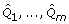F 1, v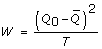, H0: Q = Q 0 v = (m - 1)(1 + r -1)2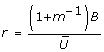B) Multivariate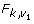H0:Q = Q 0,
k = number of parameters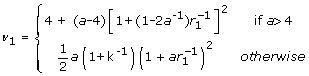where a = k(m - 1)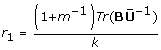C)
χ 2 statistics
w 1,..., w m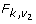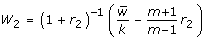k = df associated with χ 2 testsD) Likelihood Ratio χ 2 statistics
w L1,..., w Lm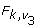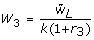k = number of parameters in fitted model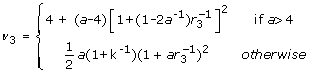where a = k(m - 1)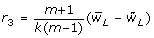1. KEY: F = value from the F-distribution, which the test statistic is compared to.
2.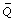= average of the m imputed data estimates.
3.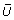= within imputation variance.
4. B = between imputation variance.
5. T = total variance for the combined MI estimate.
6. w j , j = 1,..., m = χ 2 statistics associated with testing the null hypothesis H o : Q = Q o on each imputed dataset, such that the significance level for the j thimputed dataset is P{> w j }, whereis the χ 2 value with k degrees of freedom (Rubin 1987).
7.= average of the repeated χ 2 statistics.
8.= average of the m likelihood ratio statistics, w L1,..., w Lm , evaluated using the average MI parameter estimates and the average of the estimates from a model fitted subject to the null hypothesis.